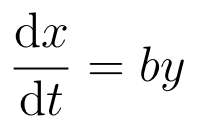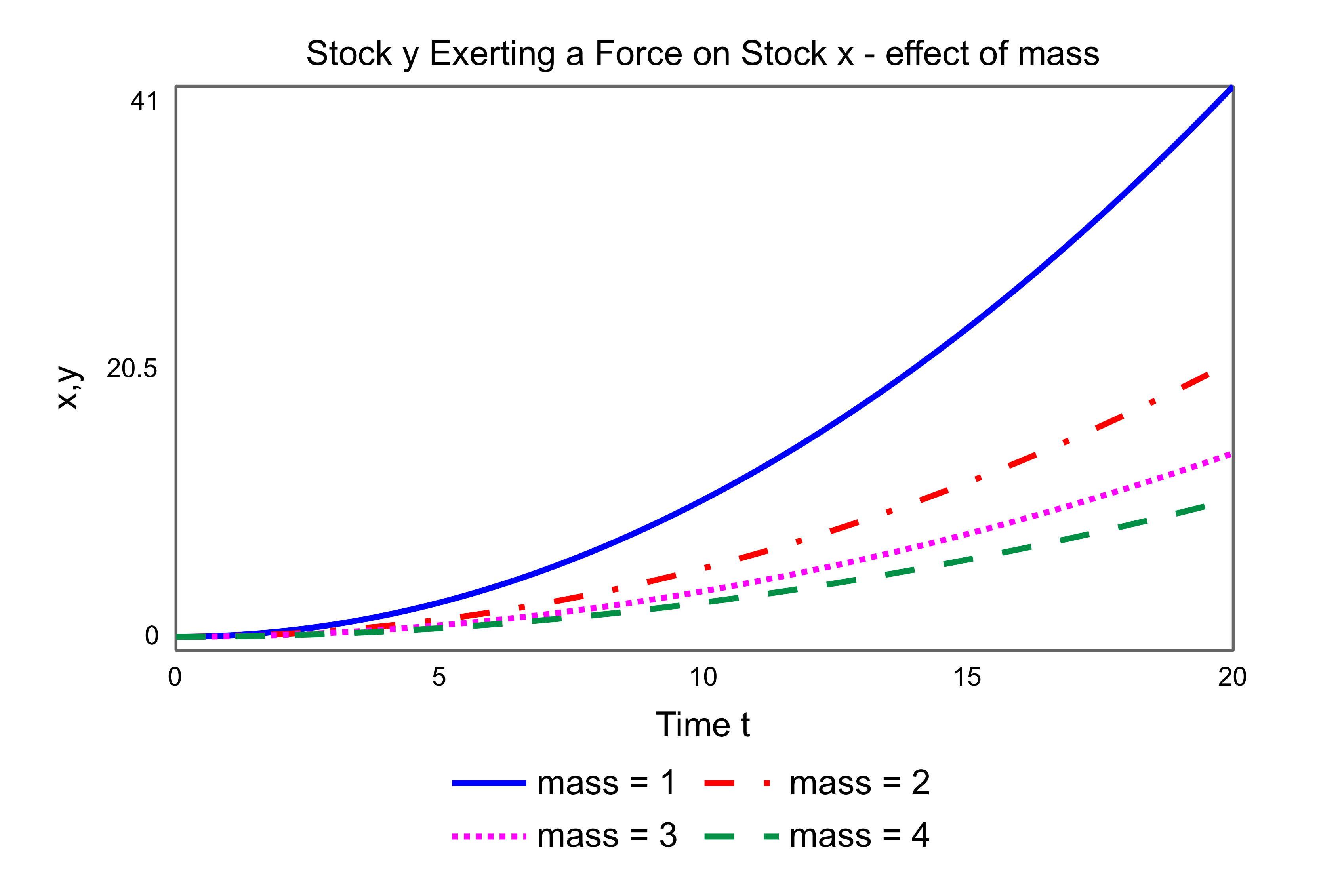# Mass

When one stock influences another, then that influence can be thought of as a force, controlled by a parameter equivalent to mass. Consider a force from one stock y to another stock x:A full explanation of this model, and the concept of force, is given on the force page.

The parameter b controls how stock x responds to changes in stock y. The larger the value of b then the more the response of x. Thus b is like the inverse of the mass of x, b = 1/m. The smaller the mass of x, the larger the value of b, the more the response of x. That is, a “lighter” stock is more responsive to a force than a “heavier” one.

The model can be expressed mathematically:Differentiating, and substituting for b, gives the acceleration of x as the equivalent of Newton’s second law of motion:Considider a constant force F for four different values of mass m. The responses of x are compared in the figure:The heavier the stock, the smaller the acceleration, i.e. the curvature in the time graph (mass = 4). The lighter the stock the more it is accelerated (mass = 1).

It is possible for a stock to be acted upon by different stocks in different units. In this case the stock could be thought of as having a different mass with repespect to each stock. In system dynamics the concept of mass is multidimensional.